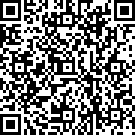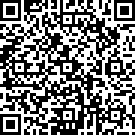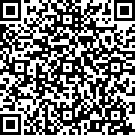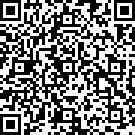无法跳出循环

0

#include <stdio.h>

{
MOUSEMSG k;
char s = {0};//储存字符串
int len = 0;
wchar_t ch;//显示字符串
settextstyle(18, 10, _T("宋体"));
int x = 0;
int num = 0;
while(1)
{
k=GetMouseMsg();
while (x < 480)//限制在文本框内输入输出
{
while(x < 10)//限制在文本框内输入输出
{
x = x + 1;
}
ch = _getwch();
if(ch == '\b')//退格键
{
clearrectangle(x+1,50+25*num,x+19,80+25*num);
x = x - 9;
continue;
}

if(ch == '\r' )  //回车键
{
break;
}
if (!(ch >= 32 && ch <= 126))//字母数字及部分符号
{
ch = 0;
}
outtextxy(x+10, 50+25*num, ch);

//将显示的字符串存储起来
s[len] = ch;
len++;
x += (ch < 256) ? 9 : 18;
if(k.x >= 500)//跳出循环    这里好像就进不去，不知道为什么
{
if(k.uMsg == WM_LBUTTONDOWN)
{
break;
}
}
}
x= 0;
num++;
if(k.x >= 500)//跳出循环
{
if(k.uMsg == WM_LBUTTONDOWN)
{
break;
}
}
}
}
1 答案
0
#include <graphics.h>
#include <stdio.h>
#include <conio.h>

void main()
{
initgraph(900, 700);
MOUSEMSG k;
char s = { 0 };//储存字符串
int len = 0;
wchar_t ch;//显示字符串
settextstyle(18, 10, _T("宋体"));
int x = 0;
int num = 0;
rectangle(100, 10, 200, 50);
bool gameover = false;
while (!gameover)
{
while (x < 480)//限制在文本框内输入输出
{
if (x < 10)//限制在文本框内输入输出
{
x = 10;
}
if (_kbhit())
{
ch = _getwch();
if (ch == '\b')//退格键
{
clearrectangle(x + 1, 50 + 25 * num, x + 19, 80 + 25 * num);
x = x - 9;
continue;
}

if (ch == '\r')  //回车键
{
break;
}
if (!(ch >= 32 && ch <= 126))//字母数字及部分符号
{
ch = 0;
}
outtextxy(x + 10, 50 + 25 * num, ch);

//将显示的字符串存储起来
s[len] = ch;
len++;
x += (ch < 256) ? 9 : 18;
}

if (MouseHit())
{
k = GetMouseMsg();
if (k.uMsg == WM_LBUTTONDOWN && k.x >= 100 && k.x <= 200 && k.y > 10 && k.y < 50)//跳出循环
{
gameover = true;
break;
}
}

}
x = 0;
num++;
}

closegraph();
}

1059085 (C语言革命0)8086730 (C语言革命1)2861971 (C语言革命2)19430220 (C语言革命6)46612969 (C语言革命7)